# The ratio 4

The ratio of two number is 5:4 if 40% of the first number is 12, what will be 50% of the second number?

x =  12

### Step-by-step explanation: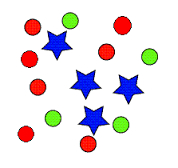Did you find an error or inaccuracy? Feel free to write us. Thank you!Tips to related online calculators
Need help to calculate sum, simplify or multiply fractions? Try our fraction calculator.
Check out our ratio calculator.
Do you have a linear equation or system of equations and looking for its solution? Or do you have a quadratic equation?

## Related math problems and questions:

• Four numbersThe first number is 50% second, the second number is 40% third, the third number is 20% of the fourth. The sum is 396. What are the numbers?
• Lions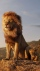If 8 lions is 40% how many would 75% be?
• The notebook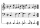After rising by 40%, the notebook cost 10.50 euros. How much did this notebook cost if it increased in price by only 20% instead of 40%?
• Alcohol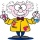6l 48% alcohol, how much 52% alcohol must be added to give 50% alcohol?
• Unknown number 24f we add 20, we get 50% of its triple. What is this unknown number?
• Two companies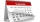The family ordered two companies to modify the garden. The first would be done in 20 days, the second would take 40% less time. How long will they work together if the second company started working when the first worked for 4 days?
• Percent calculationCalculate 8% if 44% is 32.
• PharmacyAt the pharmacy are in one container 20% solution in the second 50% solution of disinfectant. They need to prepare 4 L of 48-percent solution. What amount of solution from each container is needed to mix?
• 40% volume40% volume with 104 uph (units per labor hour) 8 people working. What is the volume?
• What percent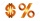What percent of 51 3/5 is 47 2/5?
• Four pupilsFour pupils divided $1485 so that the second received 50% less than the first, the third 1/2 less than a fourth, and fourth$ 154 less than the first. How much money had each of them?
• Above 100%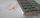Find 340 percent of the numbers 45 and 55.
• Sum of three numbersThe sum of three numbers from which second number is 20% smaller than the first number and the third number is 25% smaller than the second number is 96. Determine this numbers.
• Sales off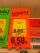shoes cost x euros. First they are discounted by 50%, and then 25% of the new amount. Determine what percentage of the total cost is cheaper.
• The manufacturer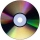The manufacturer found that 3% of the plates produced had a malfunction. Of the compliant, 75% are first and 25% second. What is the probability of producing first and second class plates?
• The number 72The number 72 increase by 25%. By how much % will you have to reduce the number you created to get the number 72 again?
• Price saleoffShoes standing y euros. At first, they were discounted by 12% and then 50% of the new amount. After this double cheapening the cost was exactly 22 euros. Determine the original price of the shoes.